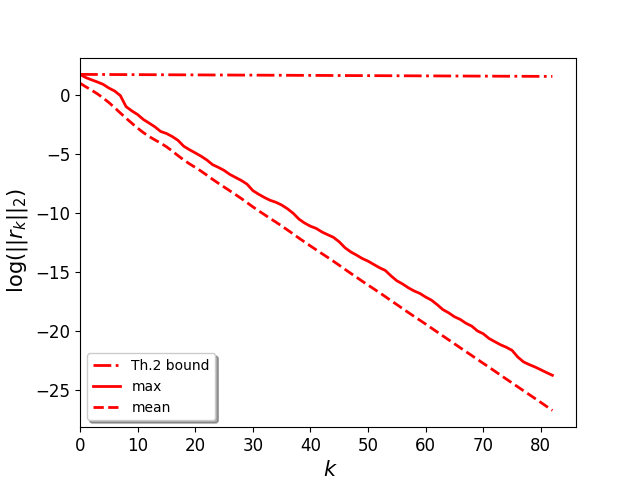# Paper accepted at IEEE Trans. on Information Theory: Recovery and convergence rate of the Frank-Wolfe Algorithm for the m-EXACT-SPARSE Problem, by F. Cherfaoui et al.Exponential convergence of the signal residual norm (reconstruction error) along iterations. Farah Cherfaoui and al. propose a theorem that bounds this residual norm.

Our paper entitled Recovery and convergence rate of the Frank-Wolfe Algorithm for the m-EXACT-SPARSE Problem by F. Cherfaoui, V. Emiya, L. Ralaivola and S. Anthoine has been accepted for a publication in the IEEE Transactions on Information Theory journal.

Abstract : We study the properties of the Frank-Wolfe algorithm to solve the m-EXACT-SPARSE reconstruction problem, where a signal y must be expressed as a sparse linear combination of a predefined set of atoms, called dictionary. We prove that when the dictionary is quasi-incoherent, then the iterative process implemented by the Frank-Wolfe algorithm only recruits atoms from the support of the signal, that is the smallest set of atoms from the dictionary that allows for a perfect reconstruction of y. We also prove that when the dictionary is quasi-incoherent, there exists an iteration beyond which the algorithm converges exponentially.